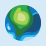# Symbolize Polyline Feature based on Point Feature Values Logic If Statements

115
1
05-12-2021 07:26 AMNew Contributor II

I have GeoEvent grabbing real-time status for point layer features. I am trying to develop a workflow that will give a related Polyline Feature a status based on the point layer features. I want this to be displayed in a WebMap or Dashboard I already have created.

Example:

Point A - Status = 0

Point B - Status = 1

Point C  Status = 0

Polyline A - Status = 1

Polyline B - Status = 0

I believe I can use IF statements to write code that has rules assigned to it that will change the status of the Polyline features based on those rules.

If Point A = 0, and Point B = 1 and Point C = 0, then Polyline A = 1

If Point A = 1, and Point B = 1 and Point C = 0, then Polyline A = 0

If Point A = 0, and Point B = 1 and Point C = 1, then Polyline A = 0

To my knowledge, ESRI does not support symbolizing layers based on ARCADE in the popups.  Any direction/help would be much appreciated.

Tags (4)
1 ReplybyEsri Contributor

You double posted 🙂

Hi, you can build/use a view to achieve what you want.  View can be created from pro or from sql.

select case
when Point A = 0, and Point B = 1 and Point C = 0 THEN Polyline A = 1
WHEN Point A = 1, and Point B = 1 and Point C = 0 THEN Polyline A = 0
ELSE Polyline A = 1

END as status,

attribute1,

attribute2,

from table a inner join table b on a.id = b.id